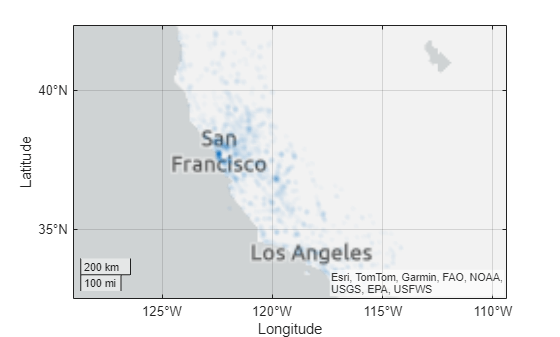# geodensityplot

Geographic density plot

## Syntax

``geodensityplot(lat,lon)``
``geodensityplot(lat,lon,weights)``
``geodensityplot(___,Name,Value)``
``geodensityplot(gx,___)``
``dp = geodensityplot(___)``

## Description

example

````geodensityplot(lat,lon)` creates a density plot in a geographic axes from locations specified (in degrees) by the coordinate vectors `lat` and `lon`. `lat` and `lon` must be the same size.```
````geodensityplot(lat,lon,weights)` specifies weights for the data points in `weights`.```
````geodensityplot(___,Name,Value)` specifies `DensityPlot` properties using one or more Name,Value pair arguments.```
````geodensityplot(gx,___)` plots in the geographic axes specified by `gx` instead of the current axes.`gx`.```
````dp = geodensityplot(___)` returns a `DensityPlot` object.```

## Examples

collapse all

Set up latitude and longitude data.

```lon = linspace(-170,170,3000) + 10*rand(1,3000); lat = 50 * cosd(3*lon) + 10*rand(size(lon));```

Specify weights for each data point.

`weights = 101 + 100*(sind(2*lon));`

Create the geographic density plot, specifying the colors used with the plot.

`geodensityplot(lat,lon,weights,'FaceColor','interp')`## Input Arguments

collapse all

Latitude coordinates of data points, specified as a real, numeric, finite vector in degrees, within the range [-90 90]. The vector can contain embedded `NaN`s. `lat` must be the same size as `lon`.

Example: `[43.0327 38.8921 44.0435]`

Data Types: `single` | `double`

Longitude coordinates in degrees, specified as a real, numeric, finite vector. The vector can contain embedded `NaN`s. `lon` must be the same size as `lat`.

Longitudes must span less than (or equal to) 360 degrees.

Example: `[-107.5556 -77.0269 -72.5565]`

Data Types: `single` | `double`

Weights assigned to data, specified as an empty array, a scalar, or a numeric array. If you specify a numeric vector, the vector must be the same length as the `lat` and `lon` vectors. The `weights` vector is typically additional data you have related to the location data in `lat` and `lon`.

Data Types: `single` | `double` | `int8` | `int16` | `int32` | `int64` | `uint8` | `uint16` | `uint32` | `uint64`

Target geographic axes, specified as a `GeographicAxes` object.1 You can modify the appearance and behavior of a `GeographicAxes` object by setting its properties. For a list of properties, see GeographicAxes Properties.

### Name-Value Arguments

Specify optional pairs of arguments as `Name1=Value1,...,NameN=ValueN`, where `Name` is the argument name and `Value` is the corresponding value. Name-value arguments must appear after other arguments, but the order of the pairs does not matter.

Before R2021a, use commas to separate each name and value, and enclose `Name` in quotes.

Example: ```dp = geodensityplot(lat,lon,weights,'FaceColor','g')```

Note

The density plot properties listed here are frequently used properties. For a complete list, see DensityPlot Properties.

Face transparency, specified as one of these values:

• Scalar in range `[0,1]` — Use uniform transparency across all the faces. A value of `1` is fully opaque and `0` is completely transparent. Values between `0` and `1` are semitransparent.

• `'interp'` — Use interpolated transparency for each face. The transparency varies across each face by interpolating the values at the vertices. Transparency values are chosen from the parent axes alphamap.

Face color, specified as `'interp'`, an RGB triplet, a hexadecimal color code, a color name, or a short name. The value `'interp'` uses interpolated coloring based on density values, where colors are chosen from the colormap of the parent axes.

RGB triplets and hexadecimal color codes are useful for specifying custom colors.

• An RGB triplet is a three-element row vector whose elements specify the intensities of the red, green, and blue components of the color. The intensities must be in the range `[0,1]`; for example,``` [0.4 0.6 0.7]```.

• A hexadecimal color code is a character vector or a string scalar that starts with a hash symbol (`#`) followed by three or six hexadecimal digits, which can range from `0` to `F`. The values are not case sensitive. Thus, the color codes `'#FF8800'`, `'#ff8800'`, `'#F80'`, and `'#f80'` are equivalent.

Alternatively, you can specify some common colors by name. This table lists the named color options, the equivalent RGB triplets, and hexadecimal color codes.

Color NameShort NameRGB TripletHexadecimal Color CodeAppearance
`'red'``'r'``[1 0 0]``'#FF0000'``'green'``'g'``[0 1 0]``'#00FF00'``'blue'``'b'``[0 0 1]``'#0000FF'``'cyan'` `'c'``[0 1 1]``'#00FFFF'``'magenta'``'m'``[1 0 1]``'#FF00FF'``'yellow'``'y'``[1 1 0]``'#FFFF00'``'black'``'k'``[0 0 0]``'#000000'``'white'``'w'``[1 1 1]``'#FFFFFF'`Here are the RGB triplets and hexadecimal color codes for the default colors MATLAB® uses in many types of plots.

`[0 0.4470 0.7410]``'#0072BD'``[0.8500 0.3250 0.0980]``'#D95319'``[0.9290 0.6940 0.1250]``'#EDB120'``[0.4940 0.1840 0.5560]``'#7E2F8E'``[0.4660 0.6740 0.1880]``'#77AC30'``[0.3010 0.7450 0.9330]``'#4DBEEE'``[0.6350 0.0780 0.1840]``'#A2142F'`Radius of influence each point has on density calculation, specified as a numeric scalar. When used in a `GeographicAxes`, the value is measured in meters.

## Output Arguments

collapse all

Geographic density plot, returned as a `DensityPlot` object.

## Tips

• To customize the geographic axes, modify geographic axes properties. For a list of properties, see GeographicAxes Properties.

• If you have Mapping Toolbox™, you can specify basemaps of your own choosing using the `addCustomBasemap` function.

• When you plot on geographic axes, the `geodensityplot` function assumes that coordinates are referenced to the WGS84 coordinate reference system. If you plot using coordinates that are referenced to a different coordinate reference system, then the coordinates may appear misaligned.

## Version History

Introduced in R2018b